# 4.1: Covalent Bonds

•• Contributed by Anonymous
• LibreTexts

Learning Objectives

• To describe how a covalent bond forms.
• To apply the octet rule to covalent compounds

You have already seen examples of substances that contain covalent bonds. One substance mentioned previously was water ($$\ce{H2O}$$). You can tell from its formula that it is not an ionic compound; it is not composed of a metal and a nonmetal. Consequently, its properties are different from those of ionic compounds.

## Electron Sharing

Previously, we discussed ionic bonding where electrons can be transferred from one atom to another so that both atoms have an energy-stable outer electron shell. Because most filled electron shells have eight electrons in them, chemists called this tendency the octet rule. However, there is another way an atom can achieve a full valence shell: atoms can share electrons.

This concept can be illustrated by using two hydrogen atoms, each of which has a single electron in its valence shell. (For small atoms such as hydrogen atoms, the valence shell will be the first shell, which holds only two electrons.) We can represent the two individual hydrogen atoms as follows: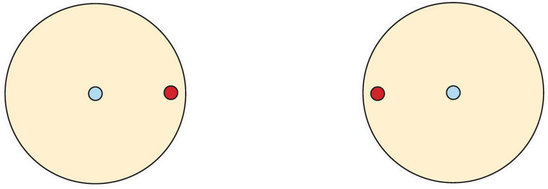In contrast, when two hydrogen atoms get close enough together to share their electrons, they can be represented as follows:By sharing their valence electrons, both hydrogen atoms now have two electrons in their respective valence shells. Because each valence shell is now filled, this arrangement is more stable than when the two atoms are separate. The sharing of electrons between atoms is called a covalent bond, and the two electrons that join atoms in a covalent bond are called a bonding pair of electrons. A discrete group of atoms connected by covalent bonds is called a molecule—the smallest part of a compound that retains the chemical identity of that compound.

Chemists frequently use Lewis diagrams to represent covalent bonding in molecular substances. For example, the Lewis diagrams of two separate hydrogen atoms are as follows: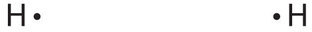The Lewis diagram of two hydrogen atoms sharing electrons looks like this:This depiction of molecules is simplified further by using a dash to represent a covalent bond. The hydrogen molecule is then represented as follows:Remember that the dash, also referred to as a single bond, represents a pair of electrons.

The bond in a hydrogen molecule, measured as the distance between the two nuclei, is about 7.4 × 10−11 m, or 74 picometers (pm; 1 pm = 1 × 10−12 m). This particular bond length represents a balance between several forces: the attractions between oppositely charged electrons and nuclei, the repulsion between two negatively charged electrons, and the repulsion between two positively charged nuclei. If the nuclei were closer together, they would repel each other more strongly; if the nuclei were farther apart, there would be less attraction between the positive and negative particles.

Fluorine is another element whose atoms bond together in pairs to form diatomic (two-atom) molecules. Two separate fluorine atoms have the following electron dot diagrams: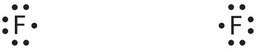Each fluorine atom contributes one valence electron, making a single bond and giving each atom a complete valence shell, which fulfills the octet rule:The circles show that each fluorine atom has eight electrons around it. As with hydrogen, we can represent the fluorine molecule with a dash in place of the bonding electrons:Each fluorine atom has six electrons, or three pairs of electrons, that are not participating in the covalent bond. Rather than being shared, they are considered to belong to a single atom. These are called nonbonding pairs (or lone pairs) of electrons.

## Covalent Bonds between Different Atoms

Now that we have looked at electron sharing between atoms of the same element, let us look at covalent bond formation between atoms of different elements. Consider a molecule composed of one hydrogen atom and one fluorine atom:Each atom needs one additional electron to complete its valence shell. By each contributing one electron, they make the following molecule: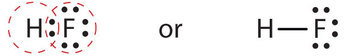In this molecule, the hydrogen atom does not have nonbonding electrons, while the fluorine atom has six nonbonding electrons (three lone electron pairs). The circles show how the valence electron shells are filled for both atoms.

Example $$\PageIndex{1}$$

Use Lewis diagrams to indicate the formation of the following:

1. Cl2
2. HBr

Solution

a. When two chlorine atoms form a chlorine molecule, they share one pair of electrons. In Cl2 molecule, each chlorine atom is surrounded by an octet number of electrons.

The Lewis diagram for a Cl2 molecule is similar to the one for F2 (shown above).b. When a hydrogen atom and a bromine atom form HBr, they share one pair of electrons. In the HBr molecule, H achieves a full valence of two electrons (duet) while Br achieves an octet. The Lewis diagram for HBr is similar to that for HF shown above.Exercise $$\PageIndex{1}$$

Draw the Lewis diagram for each compound.

1. a molecule composed of one chlorine atom and one fluorine atom
2. a molecule composed of one hydrogen atom and one iodine atom## Covalent Bonds in Larger Molecules

The formation of a water molecule from two hydrogen atoms and an oxygen atom can be illustrated using Lewis dot symbols (shown below). The structure on the right is the Lewis electron structure, or Lewis structure, for H2O. With two bonding pairs and two lone pairs, the oxygen atom has now completed its octet. Moreover, by sharing a bonding pair with oxygen, each hydrogen atom now has a full valence shell of two electrons. Chemists usually indicate a bonding pair by a single line, as shown (below).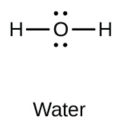Other large molecules are constructed in a similar fashion, with some atoms participating in more than one covalent bond. For example, methane ($$\ce{CH4}$$), the central carbon atom bonded to four hydrogen atoms, can be represented using either of the Lewis structures below. Again, sharing electrons between C and H atoms results in C achieving and octet while H achieving a duet number of electrons.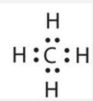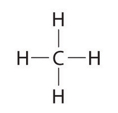## How Many Covalent Bonds Are Formed?

The number of bonds that an atom can form can often be predicted from the number of electrons needed to reach an octet (eight valence electrons). In the Lewis structure, the number of bonds formed by an element in a neutral compound is the same as the number of unpaired electrons it must share with other atoms to complete its octet of electrons. For example, each atom of a group 4A (14) element has four electrons in its outermost shell and therefore requires four more electrons to reach an octet. These four electrons can be gained by forming four covalent bonds, as illustrated here for carbon in CH4 (methane). Group 5A (15) elements such as nitrogen have five valence electrons in the atomic Lewis symbol: one lone pair and three unpaired electrons. To obtain an octet, these atoms form three covalent bonds, as in NH3 (ammonia). Oxygen and other atoms in group 6A (16) obtain an octet by forming two covalent bonds. Fluorine and the other halogens in group 7A (17) have seven valence electrons and can obtain an octet by forming one covalent bond.Typically, the atoms of group 4A form 4 covalent bonds; group 5A form 3 bonds; group 6A form 2 bonds; and group 7A form one bond. The number of electrons required to obtain an octet determines the number of covalent bonds an atom can form. This is summarized in the table below. In each case, the sum of the number of bonds and the number of lone pairs is 4, which is equivalent to eight (octet) electrons.

 Atom (Group number) Number of Bonds Number of Lone Pairs Carbon (Group 14 or 4A) 4 0 Nitrogen (Group 15 or 5A) 3 1 Oxygen (Group 16 or 6A) 2 2 Fluorine (Group 17 or 7A) 1 3

Because hydrogen only needs two electrons to fill its valence shell, it follows the duet rule. It is an exception to the octet rule. Hydrogen only needs to form one bond. This is the reason why H is always a terminal atom and never a central atom. Figure $$\PageIndex{1}$$ shows the number of covalent bonds various atoms typically form.

The transition elements and inner transition elements also do not follow the octet rule since they have d and f electrons involved in their valence shells.Figure $$\PageIndex{1}$$: How Many Covalent Bonds Are Formed? In molecules, there is a pattern to the number of covalent bonds that different atoms can form. Each block with a number indicates the number of covalent bonds formed by that atom in neutral compounds.

Example $$\PageIndex{2}$$

Examine the Lewis structure of OF2 below. Count the number of bonds formed by each element. Based on the element's location in the periodic table, does it correspond to the expected number of bonds shown in Table 4.1? Does the Lewis structure below follow the octet rule?Solution

Yes. F (group 7A) forms one bond and O (group 6A) forms 2 bonds. Each atom is surrounded by 8 electrons. This structure satisfies the octet rule.

Exercise $$\PageIndex{2}$$

Examine the Lewis structure of NCl3 below. Count the number of bonds formed by each element. Based on the element's location in the periodic table, does it correspond to the expected number of bonds shown in Table 4.1? Does the Lewis structure below follow the octet rule?Both Cl and N form the expected number of bonds. Cl (group 7A) has one bond and 3 lone pairs. The central atom N (group 5A) has 3 bonds and one lone pair. Yes, the Lewis structure of NCl3 follows the octet rule.

## Concept Review Exercises

1. How is a covalent bond formed between two atoms?

2. How does covalent bonding allow atoms in group 6A to satisfy the octet rule?

1. Covalent bonds are formed by two atoms sharing electrons.

2. The atoms in group 6A make two covalent bonds.

## Key Takeaways

• A covalent bond is formed between two atoms by sharing electrons.
• The number of bonds an element forms in a covalent compound is determined by the number of electrons it needs to reach octet.
• Hydrogen is an exception to the octet rule. H forms only one bond because it needs only two electrons.

## Exercises

1. Define covalent bond.

2. What is electron sharing?

3. Draw the Lewis diagram for the covalent bond in the H2 molecule.

4. Draw the Lewis diagram for the covalent bond in the Br2 molecule.

5. Draw the Lewis diagram for the covalent bond in the HCl molecule.

6. What is the difference between a molecule and a formula unit?

7. Why do hydrogen atoms not follow the octet rule when they form covalent bonds?

8. Draw the Lewis diagram for the covalent bonding in H2S. How many bonding electrons and nonbonding electrons are in the molecule?

9. Draw the Lewis diagram for the covalent bonding in CF4. How many bonding electrons and nonbonding electrons are in the molecule?

10. Draw the Lewis diagram for the covalent bonding in PCl3. How many bonding electrons and nonbonding electrons are in the molecule?

11. How many covalent bonds does a hydrogen atom typically form? Why?

12. How many covalent bonds does an oxygen atom typically form? Why?

13. Tellurium atoms make covalent bonds. How many covalent bonds would a tellurium atom make? Predict the formula of a compound between tellurium and hydrogen.

14. Tin atoms make covalent bonds. How many covalent bonds would a tin atom make? Predict the formula of a compound between tin and hydrogen.

15. Astatine is a synthetic element, made one atom at a time in huge “atom-smasher” machines. It is in the halogen group on the periodic table. How many covalent bonds would an atom of this element form?

16. There have been reports that atoms of element 116 (Lv) were made by smashing smaller atoms together. Using the periodic table, determine what column element 116 would be in and suggest how many covalent bonds an atom of this element would form.

1. A covalent bond is formed when two atoms share electrons.

2. Electron sharing joins two atoms in a covalent bond. This is a more stable arrangement than 2 individual atoms.

3.4.5.6. A molecule is a discrete combination of atoms; a formula unit is the lowest ratio of ions in a crystal.
7. Hydrogen atoms follow the duet rule (not the octet rule). This is because it has only one shell and this shell can only hold 2 electrons.
8.bonding electrons: 4; nonbonding electrons: 4
9.bonding electrons: 8; nonbonding electrons: 24
10.bonding electrons: 6; nonbonding electrons: 20

11. Hydrogen atoms form only one covalent bond because they have only one valence electron to pair.

12. Oxygen atoms form 2 covalent bonds because oxygen atoms have 6 valence electrons (2 lone pairs plus 2 unpaired electrons that are shared to achieve octet).

13. two; H2Te

14. four: SnH4

15. one

16. two